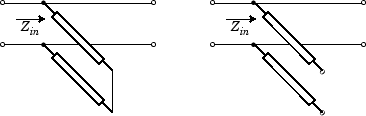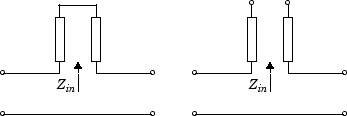rfckt.txline

General transmission line

Description

Use the txline class to represent transmission lines that are characterized by line loss, line length, stub type, and termination.

Creation

Description

example

h = rfckt.txlinereturns a transmission line object whose properties are set to their default values.

h = rfckt.txline(Name,Value) sets properties using one or more name-value pairs. For example, rfckt.txline('Z0',75) creates a transmission line object with characteristic impedance of 75 ohms. You can specify multiple name-value pairs. Enclose each property name in a quote. Properties not specified retain their default values.

Properties

expand all

Computed S-parameters, noise figure, OIP3, and group delay values, specified as rfdata.data object. This is a read-only property. For more information refer, Algorithms.

Data Types: function_handle

Frequency data for the RLCG values, specified as a M-element vector in Hz. The values must be positive and correspond to the order of loss and phase velocity values. By default, this property is empty.

Data Types: double

Interpolation method used in rfckt.rlcgline, specified as one of the following values:

MethodDescription
LinearLinear interpolation
SplineCubic spline interpolation
CubicPiecewise cubic Hermite interpolation

Data Types: char

Physical length of transmission line, specified as a scalar in meters. The default value is 0.01.

Data Types: double

Reduction in strength of signal as it travels through the transmission line, specified as a nonnegative M-element vector in decibels per meter.

Data Types: double

Object name, specified as a 1-by-N character array. This is a read-only property.

Data Types: char

Number of ports, specified as a positive integer. This is a read-only property. The default value is 2.

Data Types: double

Phase velocity or propagation velocity of a uniform plane wave on the transmission line specified as a M-element vector in meters/sec. The phase velocity values correspond to the frequency values. The default value is 299792458.

Data Types: double

Type of stub, specified as one of the following values: 'NotaStub', 'Series', 'Shunt'.

Data Types: double

Stub transmission line termination, specified as one of the following values: 'NotApplicable', 'Open', 'Short'.

Data Types: double

Characteristic impedance, specified as a vector in ohms. The default value is 50 ohms.

Data Types: double

Object Functions

 analyze Analyze RFCKT object in frequency domain calculate Calculate specified parameters for rfckt objects or rfdata objects plotyy Plot parameters of RF circuit or RF data on X-Y plane with two Y-axes circle Draw circles on Smith Chart loglog Plot specified circuit object parameters using log-log scale plot Plot circuit object parameters on X-Y plane listparam List valid parameters for specified circuit object getz0 Calculate characteristic impedance of RFCKT transmission line object semilogx Plot RF circuit object parameters using log scale for x-axis semilogy Plot RF circuit object parameters using log scale for y-axis polar Plot specified object parameters on polar coordinates smith Plot circuit object parameters on Smith chart write Write RF data from circuit or data object to file

Examples

collapse all

Transmission Line Properties

trl = rfckt.txline('Z0',75)
trl =
rfckt.txline with properties:

LineLength: 0.0100
StubMode: 'NotAStub'
Termination: 'NotApplicable'
Freq: 1.0000e+09
Z0: 75
PV: 299792458
Loss: 0
IntpType: 'Linear'
nPort: 2
AnalyzedResult: []
Name: 'Transmission Line'

Plot

f = [1e9:1.0e7:3e9];     % Simulation frequencies
analyze(trl,f);          % Do frequency domain analysis
figure
plot(trl,'s21','angle'); % Plot angle of S21Algorithms

The analyze method treats the transmission line, which can be lossy or lossless, as a 2-port linear network. It computes the AnalyzedResult property of a stub or as a stubless line using the data stored in the rfckt.txline object properties as follows:

• If you model the transmission line as a stub less line, the analyze method first calculates the ABCD-parameters at each frequency contained in the modeling frequencies vector. It then uses the abcd2s function to convert the ABCD-parameters to S-parameters.

The analyze method calculates the ABCD-parameters using the physical length of the transmission line, d, and the complex propagation constant, k, using the following equations:

$\begin{array}{l}A=\frac{{e}^{kd}+{e}^{-kd}}{2}\\ B=\frac{{Z}_{0}*\left({e}^{kd}-{e}^{-kd}\right)}{2}\\ C=\frac{{e}^{kd}-{e}^{-kd}}{2*{Z}_{0}}\\ D=\frac{{e}^{kd}+{e}^{-kd}}{2}\end{array}$

Z0 is the specified characteristic impedance. k is a vector whose elements correspond to the elements of the input vector freq. The analyze method calculates k from the specified properties as k = αa + , where αa is the attenuation coefficient and β is the wave number. The attenuation coefficient αa is related to the specified loss, α, by

${\alpha }_{a}=-\mathrm{ln}\left({10}^{\alpha /20}\right)$

The wave number β is related to the specified phase velocity, Vp, by

$\beta =\frac{2\pi f}{{V}_{p}},$

where f is the frequency range specified in the analyze input argument freq. The phase velocity Vp is derived from the rfckt.txline object properties. It is also known as the wave propagation velocity.

• If you model the transmission line as a shunt or series stub, the analyze method first calculates the ABCD-parameters at the specified frequencies. It then uses the abcd2s function to convert the ABCD-parameters to S-parameters.

When you set the StubMode property to 'Shunt', the 2-port network consists of a stub transmission line that you can terminate with either a short circuit or an open circuit as shown in the following figure.Zin is the input impedance of the shunt circuit. The ABCD-parameters for the shunt stub are calculated as:

$\begin{array}{c}A=1\\ B=0\\ C=1/{Z}_{in}\\ D=1\end{array}$

When you set the StubMode property to 'Series', the 2-port network consists of a series transmission line that you can terminate with either a short circuit or an open circuit as shown in the following figure.Zin is the input impedance of the series circuit. The ABCD-parameters for the series stub are calculated as:

$\begin{array}{c}A=1\\ B={Z}_{in}\\ C=0\\ D=1\end{array}$

 Ludwig, R. and P. Bretchko, RF Circuit Design: Theory and Applications, Prentice-Hall, 2000.